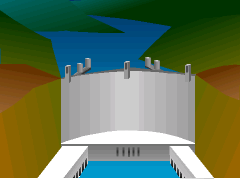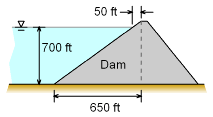Ch 2. Fluid Statics Multimedia Engineering Fluids PressureVariation PressureMeasurement HydrostaticForce(Plane) HydrostaticForce(Curved) Buoyancy
 Chapter 1. Basics 2. Fluid Statics 3. Kinematics 4. Laws (Integral) 5. Laws (Diff.) 6. Modeling/Similitude 7. Inviscid 8. Viscous 9. External Flow 10. Open-Channel Appendix Basic Math Units Basic Equations Water/Air Tables Sections Search eBooks Dynamics Fluids Math Mechanics Statics Thermodynamics Author(s): Chean Chin Ngo Kurt Gramoll ©Kurt GramollFLUID MECHANICS - CASE STUDY IntroductionHoover Dam A problem is given to students after a field trip to a dam. To simplify the analysis, assume that the dam structure is an inclined plane surface and has the dimensions shown in the figure. The water level behind the dam is 700 ft. Question Determine the magnitude and location of the resultant force exerted on the dam surface by the water. ApproachProblem Description Assume the water behind the dam has a density of 1.94 slugs/ft3, and is incompressible. Work the problem based on a unit width.

Practice Homework and Test problems now available in the 'Eng Fluids' mobile app
Includes over 250 problems with complete detailed solutions.
Available now at the Google Play Store and Apple App Store.